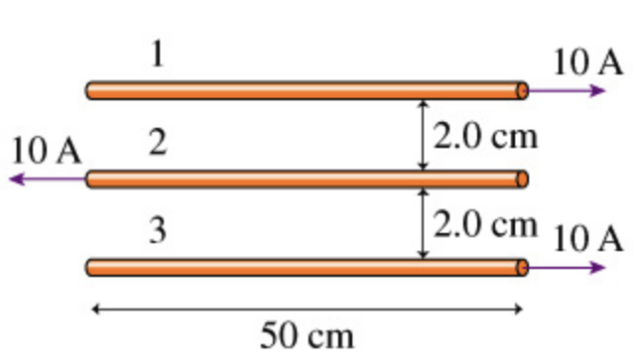In: Physics

# What is the magnitude of the net force on the first wire in (figure 1)?

Figure 1What is the magnitude of the net force on the first wire in (Figure 1)?

What is the magnitude of the net force on the second wire in (Figure 1)?

What is the magnitude of the net force on the third wire in (Figure 1)?

## Solution

##### Step #1

From the diagram, you can see that wire 1 has an attracting force from wire 3 at 0.04 m distance and a repelling force from wire 2 at 0.02 m distance.

Expression of the force is

F = µ*I1*I2*L/(2πd)

When d = 0.02 m,

F = (4*pi*10^-7*10*10*0.50) / (2*pi*0.02) = 0.0005 N

And when d = 0.04 m,

F = (4*pi*10^-7*10*10*0.50) / (2*pi*0.04) = 0.00025 N

(a) So, the net force on wire 1 = 0.005 - 0.00025 = 0.00025 N repelling, or upward.

(b) Wire 2 has equal repelling forces from wires 1 and 3, so net force = 0.

(c) By symmetry, the net force on wire 3 is the same means, 0.00025 N, but downward direction.